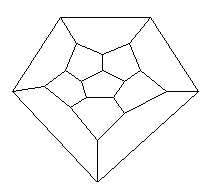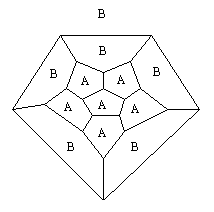## Project 5: Coloring Platonic Solids

The Platonic solids (e.g. cube, tetrahedron, octahedron, dodecahedron, and icosahedron) are very symmetric 3-dimensional polyhedra. The purpose of this project is to explore the coloring of the faces (see extensions) of these solids which takes account of their structure and symmetry.

Let us take the dodecahedron as an example. This solid has 12 faces, 20 vertices, and 30 edges. Note that it is not necessary to think of the dodecahedron as a solid in three-dimensional space for the purpose of this investigation but in terms of the diagram shown below:Consider the question of coloring the faces of this solid. Since the dodecahedron has 12 faces, consider the (non-trivial) divisors of 12: 2, 3, 4, and 6. This means that the faces of the dodecahedron can be thought of as falling in 2 groups of 6, 3 groups of 4, 4 groups of 3, or 6 groups of 2. Think of all of the faces in each group as getting the same color. Thus, one can consider coloring two groups of six faces each with a different color or 3 groups of 4 faces each with a different color.

If two faces have an edge in common, color them with different colors. (If faces meet at a vertex but do not share an edge, they can be colored the same color.)

However, one might consider other coloring rules which might be of interest. Examples include:

having a coloring where some symmetry (or all of the symmetries) of the polyhedron are "consistent with" the coloring (known as a perfect coloring when all the symmetries are "consistent" with the coloring).
having each color appear at every vertex of the solid (known as a precise coloring).

(Remark: It is possible for a coloring to be perfect but not precise, precise but not perfect, or both perfect and precise.)

As an example, consider the two-coloring of the dodecahedron with the colors A and B shown below. This coloring does not obey the traditional rule for coloring faces nor the rule that each color should appear at every vertex. However, it has the appealing property that a "symmetry" of the solid will interchange the colors. Also, a variety of symmetries will preserve the coloring. Note that the "infinite" region of the figure must receive a color and in this case it has been colored "B." (There are 11 finite faces and 1 one infinite face in this diagram. Pictures of a similar kind for the other Platonic solids will also have one "infinite" face.)Problem

Examine colorings of the Platonic solids as suggested above. (You might want to start your investigation with the tetrahedron and work your way up to the more complicated cube and octahedron, and then the dodecahedron and icosahedron.) Your goal is to enumerate all of the different possible colorings with a particular number of colors, subject to some coloring rule.

Extensions:

1. Consider the issue of coloring the vertices of these solids instead of the faces.

2. Consider the issue of coloring the edges of these solids instead of the faces.

3. Consider the issue of coloring more than one element at a time, say, the vertices and the faces or the vertices and the edges.

4. When the Platonic solids are constructed with origami paper of different but solid colors, the result is often striking. Enumerate the number of different colorings with a particular number of colors in the origami environment.

5. Extend these ideas to tilings of the plane with regular polygons.

6. Extend to other classes of polyhedra such as the Archimedean solids and deltahedra (convex solids with all faces equilateral triangles).

Reference:

1. Ore, O., Graphs and their Uses, Mathematical Association of America, Washington, 1963. (There is a new revised edition prepared by R. Wilson.)
A gentle introduction to the theory of graphs which includes a good treatment of coloring problems and the Platonic solids.

2. Barnette, D., Map Coloring, Polyhedra, and the Four-Color Problem, Mathematical Association of America, Washington, 1983.
A nice introduction to the theory of polyhedra using the theory of graphs with special attention to coloring problems.

3. Saaty, T. and P. Kainen, The Four Color Problem, McGraw Hill, New York, 1977. (Republished by Dover Press.)
A summary of the state of the art for coloring problems right after the Four Color Theorem was established by K. Appel and W. Haken.

Joseph Malkevitch
Department of Mathematics and Computing
York College (CUNY)
Jamaica, New York 11451-0001
email: malkevitch@york.cuny.edu
(Comments and results related to the project above are welcome.)

Acknowledgements
Some of this work was prepared with partial support from the National Science Foundation (Grant Number: DUE 9555401) to the Long Island Consortium for Interconnected Learning (administered by SUNY at Stony Brook, Alan Tucker, Project Director).